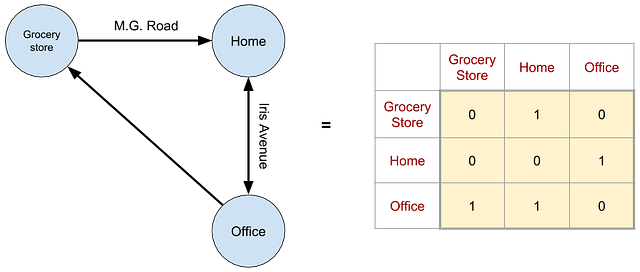# Encoding fixed length high cardinality non-numeric columns for a ML algorithm

In this post, Encoding high cardinality text data for a ML algorithm, the author compares 4 ways to encode non-numerical tabular data. This skill is quite useful and necessary to be able to use years worth tabular data in a machine learning and deep learning algorithms.

One of the ideas, Character Encoding is an interesting way to solve the problem of high cardinality. Check it out and share if you have any other ways to solve this problem.

ML algorithms work only with numerical values. So there is a need to model a problem and its data completely in numbers. For example, to run a clustering algorithm on a road network, representing the network / graph as an adjacency matrix is one way to model it.Figure 1: An simple road network represented as a Graph, where points of interest are nodes and roads connecting them are edges is shown on the left. The corresponding adjacency matrix is shown on the right

Once transformed to numbers, clustering algorithms like k-means, to identify any underlying structure, can be easily invoked like so...

Checkout the full article here including sections (with source code and illustrations) on:

1. Bucketing or Hashing
2. Character Encoding
3. Embeddings

Views: 742

Comment

Join Data Science Central